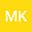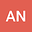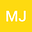Nonexistence of global solutions to wave Equations with structural damping and nonlinear memory
•••Abderrazak NABTi
Universite de Tebessa
Author ProfileMohamed Jleli
kING SAUD UNIVERSITY Riyadh, Saudi Arabia
Author Profile## Abstract

For the following wave equations with structural damping and nonlinear memory source terms $u_{tt}+(-\Delta)^{\frac{\alpha}{2}}u +(-\Delta)^{\frac{\beta}{2}}u_t =\int_{0}^{t}(t-s)^{\gamma-1} \vert u (s)\vert^{p}\,\text{d}s,$ and $u_{tt}+(-\Delta)^{\frac{\alpha}{2}}u +(-\Delta)^{\frac{\beta}{2}}u_t = \int_{0}^{t}(t-s)^{\gamma-1} \vert u_s (s)\vert^{p}\,\text{d}s,$ posed in $(x,t) \in \mathbb{R}^N \times [0,\infty)$, where $u=u(x,t)$ is real-value unknown function, $p>1$, $\alpha,\beta\in (0, 2]$, $\gamma\in (0,1)$, we prove the nonexistence of global solutions. Moreover, we give an upper bound estimate of the life span of solutions.